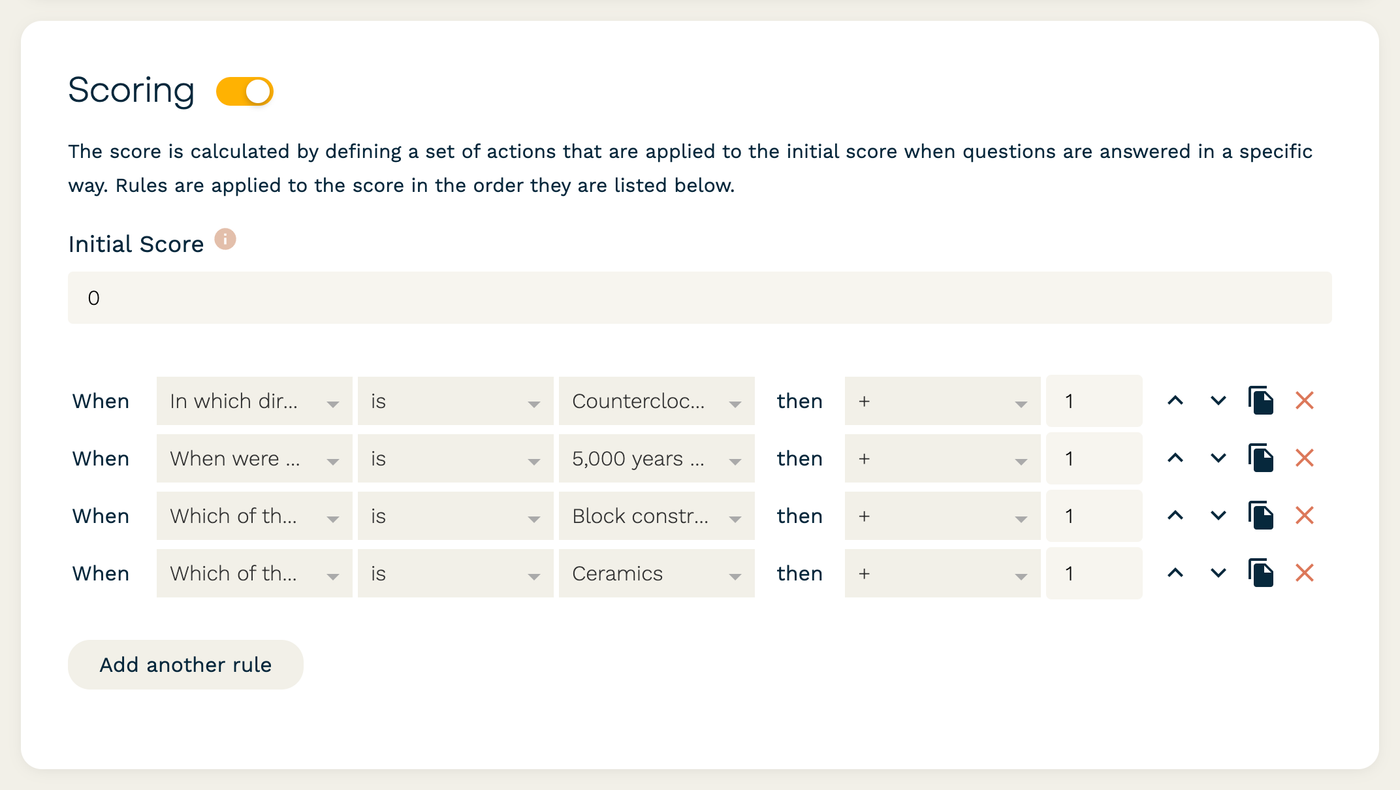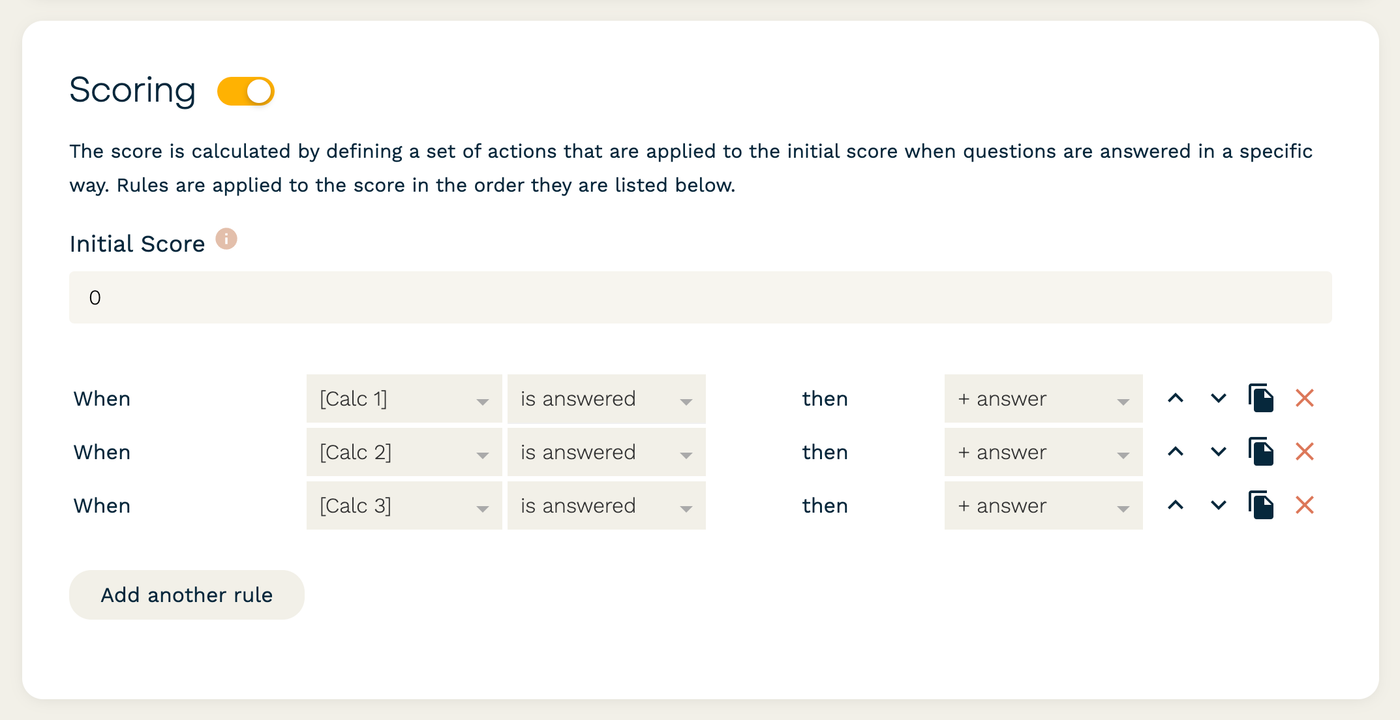It is not uncommon that throughout a quiz or test we want the submitter to view how they scored on a previous section. We can display a user's current score at different points of the form using answer piping, or we can use calculations to find and show the points from different sections separately. Although Scoring is available on all plans, both answer piping and calculations are only available on Pro or Agency.

## Display a running total

Paperform's Scoring feature allows you to assign a certain number of points to each answer (positive, negative, or even multipliers) and keep track of a single, total score. This can be set up under Configure → Form Behaviour → Scoring.On the Pro and Agency plan, you can type `{{ score }}` directly onto the form page wherever you would like the current number of points to appear. This could be used to show the running total on different pages of a longer quiz.## Calculate a separate score for each section

Instead of showing the grand total each time, you might want to determine the points for each section separately. Scoring only returns a single, running total, but you can use custom calculation fields instead to add up the points manually for each group of questions.

There are many ways to go about this, but the calculation for a specific section might look something like this:

``````IF( {{ question_1 }} == "Correct answer to Q1", 1, 0 )
+ IF( {{ question_2 }} == "Correct answer to Q2", 1, 0 )
+ IF( {{ question_3 }} == "Correct answer to Q3", 1, 0 );
``````

By creating a separate calculation for each section, the totals can be displayed on their own in different parts of the form, as well as coming through as individual values in the submission results. To show these totals on the form, you could make each calculation field visible, or you could use answer piping to display each result wherever you like.

If you still need to determine a total score from all sections, consider adding the totals from your calculations together under the Scoring menu as a shortcut. The result from one calculation cannot be used in another calculation, but they can all be added to the total Score.Calculations can get a bit tricky, so feel free to reach out to the support team in the chat box or shoot us an email at support@paperform.co if you get stuck.

## Gotchas

• You can add calculation results to the total Score, but you cannot pull the `{{ score }}` into a calculation, nor can you pull the answer from one calculation field into another.